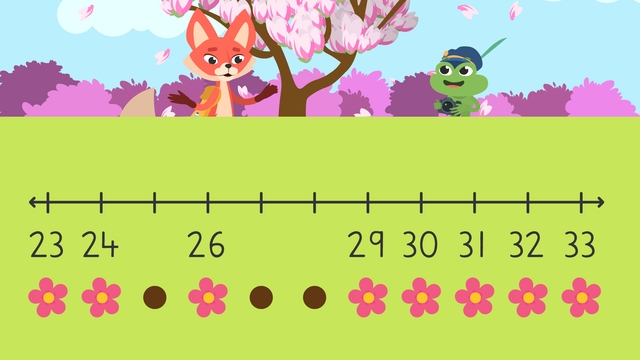# Find the Missing Number on a Number Line

Content Find the Missing Number on a Number LineRating

Ø 4.0 / 2 ratings
The authorsTeam Digital
Find the Missing Number on a Number Line
CCSS.MATH.CONTENT.2.OA.A.1

## Missing Numbers on a Number Line

In this video Skylar and Henry are going on their annual forest hike! They decide to follow a flower trail to see where it will lead them, but there’s a problem, some of the flowers are missing! They need to learn about missing numbers on a number line while following the trail. Watch this video to learn more about how to find missing addends with a number line.

## How Do You Find the Missing Number in a Number Line?

Let’s repeat the definition of a number line:

A number line is a diagram in which numbers are represented as points on a line. They count by a specific number, sometimes by ones, twos, fives. or even tens.

But, what happens when a number is missing? Follow these steps to find missing numbers on a number line:

Step # What to do
1 Look at the whole number line.
2 Look for the number line pattern –
What the number line is counting by.
3 Count using this pattern to find the
missing number on the number line pattern.
4 Reread the number line to make sure it is correct.

## Missing Numbers on Number Lines – Practice

How can you figure out missing numbers using patterns on a number line? Let’s practice filling in the missing numbers on a number line! Our number line includes numbers from 35 to 70.Can you figure out which numbers are missing from this number line? Remember our steps to find missing numbers on number lines.Let’s look at the pattern on the number line. The pattern shows incremental steps of +5! Knowing this, we can figure out the missing numbers by skip counting from the last known number. This number line is missing the numbers forty-five and fifty-five!

## Missing Numbers Number Line – Summary

When solving for missing numbers on a number line;

• First: look at the whole number line.

• Then: look for the missing number line pattern – what the number line is counting by.

• Next: count using this pattern to find the missing number!

• Finally: reread the number line again to make sure it is correct.

Looking for extra practice? After watching this video, check out the interactive exercises and worksheets on missing numbers and addends in number lines.

## Find the Missing Number on a Number Line exercise

Would you like to apply the knowledge you’ve learned? You can review and practice it with the tasks for the video Find the Missing Number on a Number Line.
• ### Number line patterns.

Hints

Here is an example of counting by ones:

12, 13, 14, 15

Here is an example of counting by fives:

30, 35, 40, 45

Here is an example of counting by tens:

40, 50, 60, 70

Solution

The number line patterns count by ones, fives, tens, and fives.

• ### Skylar and Henry go on a hike.

Hints

The first step when a number is missing on a number line is to look at the whole number line.

The second step when a number is missing on a number line is to look for the pattern or what the number line is counting by.

The third step when a number is missing on a number line is to count using this pattern to find the missing number.

The fourth step when a number is missing on a number line is to reread the number line again to make sure it is correct.

Solution

The number line counts by ones.

• The missing numbers are 46, 49, and 51.
• ### Help Skylar and Henry find the trail.

Hints

What is the pattern counting by?

Here is an example of counting by fives:

15, 20, 25, 30

What comes after 65 when counting by fives? What comes after 80 when counting by fives?

Solution

The number line counts by fives.

• The missing numbers are 70 and 85.
• ### Count the flowers.

Hints

What is the pattern counting by?

Here is an example of counting by tens:

20, 30, 40, 50

What comes after 60 when counting by tens? What comes after 90 when counting by tens?

Solution

The number line counts by tens.

• The missing numbers are 70 and 100.
• ### What are the steps of finding a missing number on a number line?

Hints

The image provides an example of the first step of finding a missing number on a number line.

The image provides an example of the second step of finding a missing number on a number line.

The image provides an example of the third step of finding a missing number on a number line.

Solution

4 steps when a number is missing on a number line:

1. Look at the whole number line.
2. Look for the pattern or what the number line is counting by.
3. Count using this pattern to find the missing number.
4. Reread the number line again to make sure it is correct.
• ### Missing numbers.

Hints

What is the pattern counting by?

Count back by tens from 90 on the number line to find the initial missing number.

Solution

The number line counts by tens.

• Count back by tens on the number line from 90 to find the initial missing number, 80.
• The missing numbers are 80, 110, and 140.